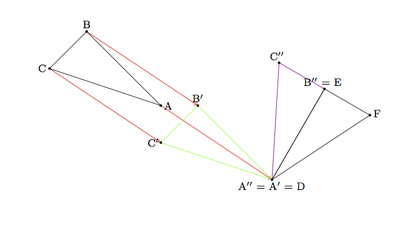# Properties of Congruent Triangles

Alignments to Content Standards: G-CO.B.7

Below is a picture of two triangles:1. Suppose there is a sequence of rigid motions which maps $\triangle ABC$ to $\triangle DEF$. Explain why corresponding sides and angles of these triangles are congruent.
2. Suppose instead that corresponding sides and angles of $\triangle ABC$ and $DEF$ are congruent. Show that there is a sequence of rigid motions which maps $\triangle ABC$ to $\triangle DEF$.

## IM Commentary

The goal of this task is to understand how congruence of triangles, defined in terms of rigid motions, relates to the corresponding sides and angles of these triangles. In particular, there is a sequence of rigid motions mapping one triangle to another if and only if these two triangles have congruent corresponding sides and angles.

One of the advantages to working with the rigid motion definition of congruence is that it allows relatively quick and clean proofs of the basic triangle congruence criteria (see tasks illustrating G-CO.8 for more details). These congruence criteria are at the heart of Euclidean geometry but providing good proofs either requires a lot of work building up from basic axioms (as is done in college modern geometry courses) or requires use of visual intuition and unstated hypotheses (as was done in Euclid's time). The properties of transformations, taken for granted in this approach to geometry, serve to make these unstated hypotheses explicit. In addition, working with transformations helps to build visual intuition and to identify important structure, namely symmetry, in nature and art.

The main mathematical practice closely associated with work on this task is MP2, Reason Abstractly and Quantitatively. Students will need to keep track of what is given and what needs to be shown since these two trade places in parts (a) and (b). In addition, they will need to match their visual ideas for how to show the triangle congruence in part (a) with the mathematical definitions of the different transformations (translations, rotations, and reflections) in part (b).

## Solution

1. The fundamental property of rigid motions of the plane is that they do not change angle measurements or side lengths. If there is a rigid transformation which maps $\triangle ABC$ to $\triangle DEF$ this means that

\begin{align} |AB| &= |DE|, \\ |AC| &= |DF|, \\ |BC| &= |EF|, \\ m(\angle A) &= m(\angle D),\\ m(\angle B) &= m(\angle E), \\ m(\angle C) &= m(\angle F). \end{align}

In other words, corresponding parts of congruent triangles are congruent.

2. We work with the given example of two triangles $ABC$ and $DEF$ with congruent corresponding sides and angles but the method presented here will work for any pair of triangles with congruent corresponding angles and sides: we have made indications throughout how the given construction would apply in general. We can choose a translation that maps $A$ to $D$ while preserving all side lengths and angles of $\triangle ABC$. The picture below shows the image of $\triangle ABC$ after this translation, denoted $\triangle A^\prime B^\prime C^\prime$:Note that if $A = D$ for the original pair of triangles then this first step is not necessary. For our next rigid motion, we would like to fix $A^\prime = D$ and match up a pair of sides of $\triangle A^\prime B^\prime C^\prime$ and $\triangle DEF$. One way to do this is to take a rotation about $A^\prime,$ through angle $B^\prime A^\prime E$, which moves $\overrightarrow{A^\prime B^\prime}$ to $\overrightarrow{DE}$ as pictured below. The new rotated triangle is denoted $A^{\prime \prime}B^{\prime \prime}C^{\prime \prime}$:Both the translation and the rotation preserve segment lengths and since $|AB| = |DE|$ by hypothesis, this means that $|A^{\prime \prime}B^{\prime \prime}| = |DE|$. Since the rays $\overrightarrow{A^\prime B^\prime}$ and $\overrightarrow{DE}$ have the same vertex and point in the same direction, this means that $B^{\prime \prime} = E$ as in the picture above. Now our triangles $A^{\prime \prime}B^{\prime \prime}C^{\prime \prime}$ share a side. If $C^{\prime \prime}$ is on the same side of $\overleftrightarrow{DE}$ as $F$ (this is not the case for our triangles) then we can show that $\triangle DEF$ and $\triangle A^{\prime \prime}B^{\prime \prime}C^{\prime \prime}$ are the same triangle: to see why, note that $\angle EDF$ and $\angle B^{\prime \prime}A^{\prime \prime}C^{\prime \prime}$ are the same since they share $\overrightarrow{DE}$, lie on the same side of $\overleftrightarrow{DE}$, and have the same measure . We also know that $|A^{\prime \prime}C^{\prime \prime}| = |DF|$ since rigid motions preserve side lengths. We conclude that $C^{\prime \prime} = F$ and, when $C^{\prime \prime}$ lies on the same side of $\overleftrightarrow{DE}$ as $F$, we have established that our translation and rotation map $\triangle ABC$ to $\triangle DEF$.

In our picture, $C^{\prime \prime}$ does not lie on the same side of $\overleftrightarrow{DE}$ as $F$, so we must reflect $\triangle A^{\prime \prime}B^{\prime \prime}C^{\prime \prime}$ about $\overleftrightarrow{DE}$. This will preserve ray $\overrightarrow{DE}$ and the image of $C^{\prime \prime}$ will be on the same side of $\overleftrightarrow{DE}$ as $F$. We can then apply the argument above to show that $\triangle ABC$ has been mapped to $\triangle DEF$ after this reflection.